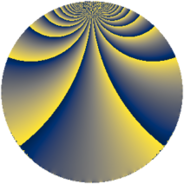Properties

 Label 115.3.iLevel $115$ Weight $3$ Character orbit 115.i Rep. character $\chi_{115}(14,\cdot)$ Character field $\Q(\zeta_{22})$ Dimension $220$ Newform subspaces $1$ Sturm bound $36$ Trace bound $0$

Related objects

Defining parameters

 Level: $$N$$ $$=$$ $$115 = 5 \cdot 23$$ Weight: $$k$$ $$=$$ $$3$$ Character orbit: $$[\chi]$$ $$=$$ 115.i (of order $$22$$ and degree $$10$$) Character conductor: $$\operatorname{cond}(\chi)$$ $$=$$ $$115$$ Character field: $$\Q(\zeta_{22})$$ Newform subspaces: $$1$$ Sturm bound: $$36$$ Trace bound: $$0$$

Dimensions

The following table gives the dimensions of various subspaces of $$M_{3}(115, [\chi])$$.

Total New Old
Modular forms 260 260 0
Cusp forms 220 220 0
Eisenstein series 40 40 0

Trace form

 $$220 q + 26 q^{4} - 11 q^{5} - 14 q^{6} + 32 q^{9} + O(q^{10})$$ $$220 q + 26 q^{4} - 11 q^{5} - 14 q^{6} + 32 q^{9} - 11 q^{10} - 22 q^{11} - 22 q^{14} - 88 q^{15} - 142 q^{16} - 22 q^{19} - 99 q^{20} - 22 q^{21} - 88 q^{24} + 17 q^{25} + 34 q^{26} + 92 q^{29} + 341 q^{30} - 152 q^{31} - 264 q^{34} - 13 q^{35} - 62 q^{36} - 118 q^{39} - 11 q^{40} - 80 q^{41} - 242 q^{44} + 226 q^{46} + 90 q^{49} - 211 q^{50} - 22 q^{51} + 658 q^{54} - 565 q^{55} + 770 q^{56} - 172 q^{59} - 891 q^{60} + 286 q^{61} - 474 q^{64} - 242 q^{65} - 44 q^{66} - 288 q^{69} + 790 q^{70} - 210 q^{71} + 506 q^{74} + 804 q^{75} - 2376 q^{76} + 462 q^{79} + 2398 q^{80} - 2408 q^{81} + 1034 q^{84} + 1197 q^{85} - 1518 q^{86} - 22 q^{89} + 154 q^{90} - 210 q^{94} - 338 q^{95} + 2772 q^{96} + 1628 q^{99} + O(q^{100})$$

Decomposition of $$S_{3}^{\mathrm{new}}(115, [\chi])$$ into newform subspaces

Label Dim. $$A$$ Field CM Traces $q$-expansion
$a_{2}$ $a_{3}$ $a_{5}$ $a_{7}$
115.3.i.a $220$ $3.134$ None $$0$$ $$0$$ $$-11$$ $$0$$